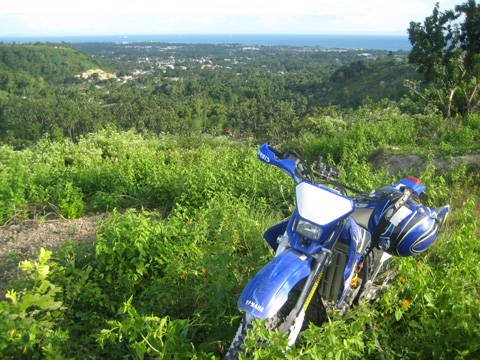Error !! Can't find data file : \$data_file.
"; exit; } else { if (\$max_record_in_data_file != "0") { \$f = file(\$data_file); rsort(\$f); \$j = count(\$f); if (\$j > \$max_record_in_data_file) { \$rf = fopen(\$data_file,"w"); if (strtoupper(\$os) == "UNIX") { if (flock(\$rf,LOCK_EX)) { for (\$i=0; \$i<\$max_record_in_data_file; \$i++) { fwrite(\$rf,\$f[\$i]); } flock(\$rf,LOCK_UN); } } else { for (\$i=0; \$i<\$max_record_in_data_file; \$i++) { fwrite(\$rf,\$f[\$i]); } } fclose(\$rf); } } } session_start(); \$newline = (strtoupper(\$os) == "WIN") ? "\r\n" : "\n"; switch (\$do) { case "": \$record = file(\$data_file); rsort(\$record); \$jmlrec = count(\$record); ?> Schedule your ride now!

# Extreme Contact# We want to hear from you!

Would you like more information about one of our tours? Have a question that the site can't answer for you? Perhaps you want to suggest a tour for us? Please drop us a line, we'd love to hear from you!

# Feedback

'; } \$w = 0; //--Color for (\$i=0; \$i<\$max_entry_per_page; \$i++) { \$nomrec++; \$no++; //\$no--; \$recno = \$nomrec-1; if (isset(\$record[\$recno])) { \$row = explode("|~|",\$record[\$recno]); if (\$w==0) { \$warna = \$table_content_1a; \$warna2 = \$table_content_1b; \$w=1; } else { \$warna = \$table_content_2a; \$warna2 = \$table_content_2b; \$w=0; } echo "'; echo ""; } //--end if } //--end for echo "'; ?>
There are no entries yet.
\$no "; echo "
 \$row \$row "; if (trim(\$row) != "") { echo ""; } if (trim(\$row) != "" && trim(\$row) != "http://") { if (ereg("^http://", trim(\$row))) echo ""; else echo ""; } echo '
'; echo "
 ".stripslashes(\$row)."
"; echo '"; if (\$jml_page > 1) { if (\$page != 1) echo "[Top] "; else echo '[Top] '; echo 'Page # '; if (\$jml_page > 10) { if (\$page < 5) { \$start = 1; \$stop = 10; } elseif (\$jml_page - \$page < 5) { \$start = \$jml_page - 9; \$stop = \$jml_page; } else { \$start = \$page-4; \$stop = \$page+5; } if (\$start != 1) echo '... '; for (\$p=\$start; \$p<=\$stop; \$p++) { if (\$p == \$page) echo "\$p  "; else echo "\$p  "; } if (\$stop != \$jml_page) echo '... '; echo "of \$jml_page "; } else { for (\$p=1; \$p<=\$jml_page; \$p++) { if (\$p == \$page) echo "\$p  "; else echo "\$p  "; } } if (\$page != \$jml_page) echo "[Bottom]"; else echo '[bottom]'; } else echo 'Page #1 of 1'; echo '

# We want to hear from you

Would you like more information about one of our tours? Have a question that the site can't answer for you? Perhaps you want to suggest a tour for us? Please drop us a line, we'd love to hear from you!

Enjoyed your ride or your visit to our website? Leave us some feedback here.
To inquire about an upcoming ride please click on the CONTACT button to the left.

Thanks!

 *Name : Email : Website : *Comment : * Required field Verification Code : Please retype this code below :70) \$vname = substr(\$vname,0,70); if (strlen(\$vemail) > 100) \$vemail = substr(\$vemail,0,100); if (strlen(\$vurl) > 150) \$vurl = substr(\$vurl,0,150); \$_SESSION['name'] = \$vname; \$_SESSION['email'] = \$vemail; \$_SESSION['url'] = \$vurl; \$_SESSION['comment'] = stripslashes(\$vcomment); if (\$vname == "" || \$vcomment == "") { input_err("You may left some fields."); } if (\$vemail != "" && !preg_match("/([\w\.\-]+)(\@[\w\.\-]+)(\.[a-z]{2,4})+/i", \$vemail)) { input_err("Invalid email address."); } if (\$vurl != "" && strtolower(\$vurl) != "http://") { if (!preg_match ("#^http://[_a-z0-9-]+\\.[_a-z0-9-]+#i", \$vurl)) { input_err("Invalid URL format."); } } \$test_comment = preg_split("/[\s]+/",\$vcomment); \$jmltest = count(\$test_comment); for (\$t=0; \$t<\$jmltest; \$t++) { if (strlen(trim(\$test_comment[\$t])) > 70) { input_err("Invalid word found on your entry : ".stripslashes(\$test_comment[\$t])); } } if (isset(\$_SESSION['add']) && \$_SESSION['add'] >= \$max_entry_per_session) { input_err("Sorry, only \$max_entry_per_session message(s) allowed per session.",false); } elseif (!isset(\$_SESSION['add'])) { exit; } if (\$vsecc != \$_SESSION['secc'] && strtoupper(\$imgcode) == "YES") { input_err("Invalid verification code"); } //--only 2000 characters allowed for comment, change this value if necessary \$maxchar = 2000; if (strlen(\$vcomment) > \$maxchar) \$vcomment = substr(\$vcomment,0,\$maxchar)."..."; \$idx = date("YmdHis"); \$tgl = date("F d, Y - h:i A"); \$vname = str_replace("<","<",\$vname); \$vname = str_replace(">",">",\$vname); \$vname = str_replace("~","-",\$vname); \$vname = str_replace("\"",""",\$vname); \$vcomment = str_replace("<","<",\$vcomment); \$vcomment = str_replace(">",">",\$vcomment); \$vcomment = str_replace("|","",\$vcomment); \$vcomment = str_replace("\"",""",\$vcomment); \$vurl = str_replace("<","",\$vurl); \$vurl = str_replace(">","",\$vurl); \$vurl = str_replace("|","",\$vurl); \$vemail = str_replace("<","",\$vemail); \$vemail = str_replace(">","",\$vemail); \$vemail = str_replace("|","",\$vemail); if (strtoupper(\$os) == "WIN") { \$vcomment = str_replace(\$newline,"
",\$vcomment); \$vcomment = str_replace("\r","",\$vcomment); \$vcomment = str_replace("\n","",\$vcomment); } else { \$vcomment = str_replace(\$newline,"
",\$vcomment); \$vcomment = str_replace("\r","",\$vcomment); } if (isset(\$_SERVER['HTTP_X_FORWARDED_FOR']) && eregi("^[0-9]{1,3}\\.[0-9]{1,3}\\.[0-9]{1,3}\\.[0-9]{1,3}\$",\$_SERVER['HTTP_X_FORWARDED_FOR'])) { \$ipnum = \$_SERVER['HTTP_X_FORWARDED_FOR']; } else { \$ipnum = getenv("REMOTE_ADDR"); } \$newdata = "|~|\$idx|~|\$tgl|~|\$vname|~|\$vemail|~|\$vcomment|~|\$vurl|~|\$ipnum|~|"; \$newdata = stripslashes(\$newdata); \$newdata .= \$newline; if (!is_spam(\$newdata)) { \$tambah = fopen(\$data_file,"a"); if (strtoupper(\$os)=="UNIX") { if (flock(\$tambah,LOCK_EX)) { fwrite(\$tambah,\$newdata); flock(\$tambah,LOCK_UN); } } else { fwrite(\$tambah,\$newdata); } fclose(\$tambah); //--send mail if (strtoupper(\$notify) == "YES") { \$msgtitle = "Someone signed your guestbook"; \$vcomment = str_replace(""","\"",\$vcomment); \$vcomment = stripslashes(\$vcomment); \$vcomment = str_replace("
","\n",\$vcomment); \$msgcontent = "Local time : \$tgl\n\nThe addition from \$vname :\n----------------------------\n\n\$vcomment\n\n-----End Message-----"; @mail(\$admin_email,\$msgtitle,\$msgcontent,"From: \$vemail\n"); } //--clear session \$_SESSION['name'] = ""; \$_SESSION['email'] = ""; \$_SESSION['url'] = "http://"; \$_SESSION['comment'] = ""; \$_SESSION['add']++; \$_SESSION['secc'] = ""; redir(\$self,"Thank you, your entry has been added."); } else { redir(\$self,"Sorry, your entry can't be added into the guestbook."); } break; case "del": \$record = file(\$data_file); \$jmlrec = count(\$record); for (\$i=0; \$i<\$jmlrec; \$i++) { \$row = explode("|~|",\$record[\$i]); if (\$id == \$row) { ?> Delete record
Delete Confirmation

 - IP :